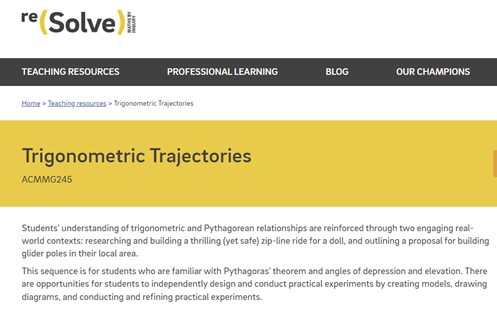Go to website

# Trigonometric trajectories

Students’ understanding of trigonometric and Pythagorean relationships are reinforced through two engaging real-world contexts. This sequence is for students who are familiar with Pythagoras’ theorem and angles of depression and elevation. There are opportunities for students to independently design and conduct practical experiments by creating models, drawing diagrams, and conducting and refining practical experiments.

Year level(s) Year 10
Audience Teacher
Format Web page
Teaching strategies and pedagogical approaches Mathematics investigation
Keywords Angle, Length, Height, Tangent, Cosine, Sine, Trigonometry, Depression, Elevation

## Curriculum alignment

Curriculum connections Critical and creative thinking
Strand and focus Space
Topics Pythagoras and trigonometry
AC: Mathematics (V9.0) content descriptions
AC9M10M03

Solve practical problems applying Pythagoras' theorem and trigonometry of right-angled triangles, including problems involving direction and angles of elevation and depression

Numeracy progression Understanding geometric properties (P7)
Understanding units of measurement (P10)